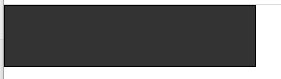# canvas基础简单易懂教程（完结，多图）# Canvas学习

canvas 读音 /ˈkænvəs/, 即kæn və s(看我死).

## 一、 Canvas概述

canvas是用来绘制图形的.它可以用于动画、游戏画面、数据可视化、图片编辑以及实时视频处理等方面。

canvas是HTML5提出的新标签，彻底颠覆了Flash的主导地位。无论是广告、游戏都可以使用canvas实现。

Canvas 是一个轻量级的画布, 我们使用Canvas进行JS的编程，不需要增加额外的组件，性能也很好，不卡顿，在手机中也很流畅。

### 1.1 Hello world

``````<canvas width="500" height="500">
当前的浏览器版本不支持，请升级浏览器
</canvas>
``````

canvas的标签属性只有两个，width和height，表示的是canvas画布的宽度和高度，不要用css来设置，而是用属性来设置，画布会失真变形。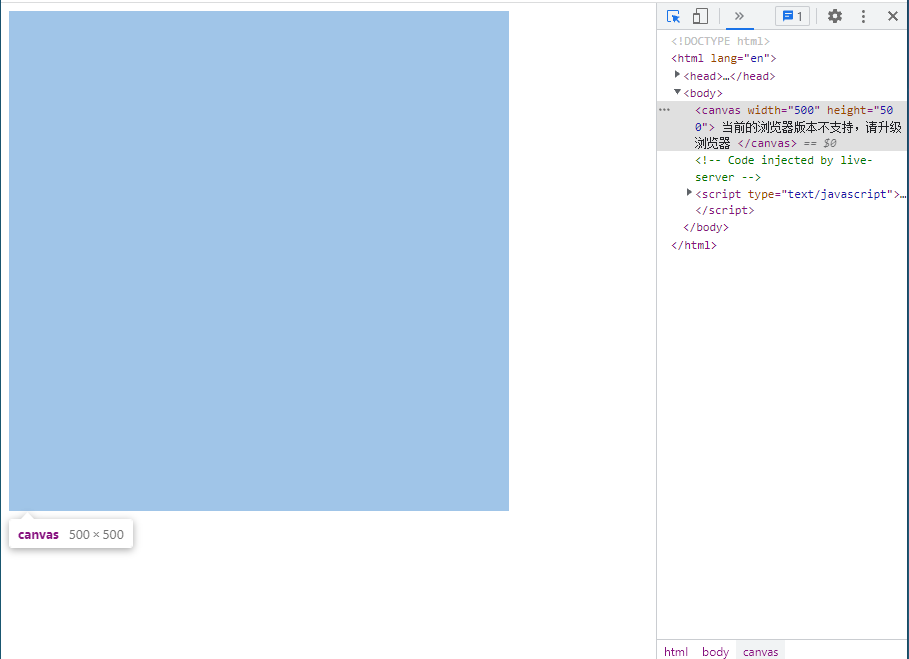``````// 得到canvas的画布
const myCanvas:HTMLCanvasElement = document.getElementById("main_canvas") as HTMLCanvasElement// 返回某种类型的HTMLElement

// 得到画布的上下文，上下文有两个，2d的上下文和3d的上下文
// 所有的图像绘制都是通过ctx属性或者是方法进行设置的，和canvas标签没有关系了
const ctx = myCanvas.getContext("2d")
if(ctx !== null) {
// 设置颜色
ctx.fillStyle = 'green'
// 绘制矩形
ctx.fillRect(100, 100, 200, 50)
}
``````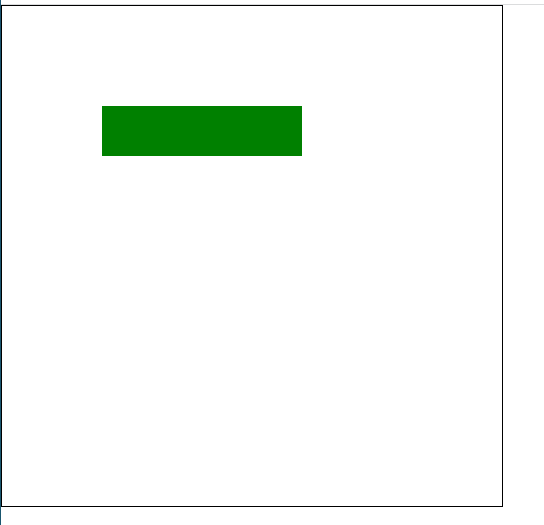### 1.3 Canvas的动画思想

canvas上画布的元素，就被像素化了，所以不能通过style.left方法进行修改，而且必须要重新绘制。

``````// 得到画布
const myCanvas:HTMLCanvasElement = document.getElementById("main_canvas") as HTMLCanvasElement

// 获取上下文
const ctx = myCanvas.getContext("2d")

if(ctx !== null) {
// 设置颜色
ctx.fillStyle = "blue"
// 初始信号量
let left:number = -200
// 动画过程
setInterval(() => {
// 清除画布,0,0代表从什么位置开始,600,600代表清除的宽度和高度
ctx.clearRect(0,0,600,600)
// 更新信号量
left++
// 如果已经走出画布，则更新信号量为初始位置
if(left > 600) {
left = -200
}
ctx.fillRect(left, 100, 200, 200)
},10)
}
``````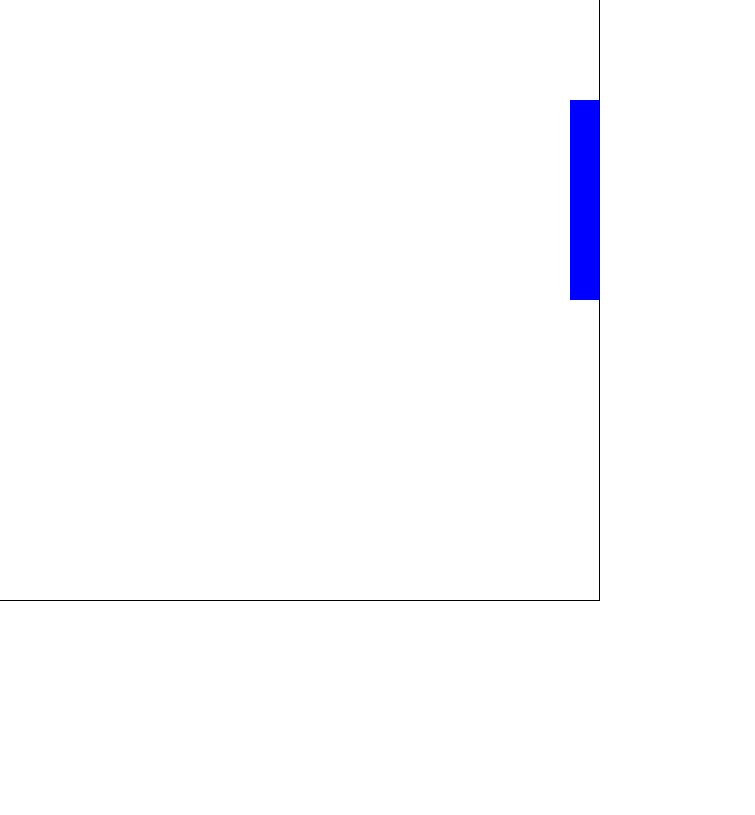### 1.4 面向对象思维实现canvas动画

``````// 得到画布
const myCanvas:HTMLCanvasElement = document.getElementById("main_canvas") as HTMLCanvasElement

// 获取上下文
const ctx = myCanvas.getContext("2d")

class Rect {
// 维护状态
constructor(
public x: number,
public y: number,
public w: number,
public h: number,
public color: string
) {
}
// 更新的方法
update() {
this.x++
if(this.x > 600) {
this.x = -200
}
}
// 渲染
render(ctx: CanvasRenderingContext2D) {
// 设置颜色
ctx.fillStyle = this.color
// 渲染
ctx.fillRect(this.x, this.y, this.w, this.h)
}
}

// 实例化
let myRect1: Rect = new Rect(-100, 200, 100, 100, 'purple')
let myRect2: Rect = new Rect(-100, 400, 100, 100, 'pink')

// 动画过程

// 更新的办法
setInterval(() => {
// 清除画布,0,0代表从什么位置开始,600,600代表清除的宽度和高度
if(ctx !== null) {
// 清屏
ctx.clearRect(0,0,600,600)
// 更新方法
myRect1.update()
myRect2.update()
// 渲染方法
myRect1.render(ctx)
myRect2.render(ctx)
}
},10)
``````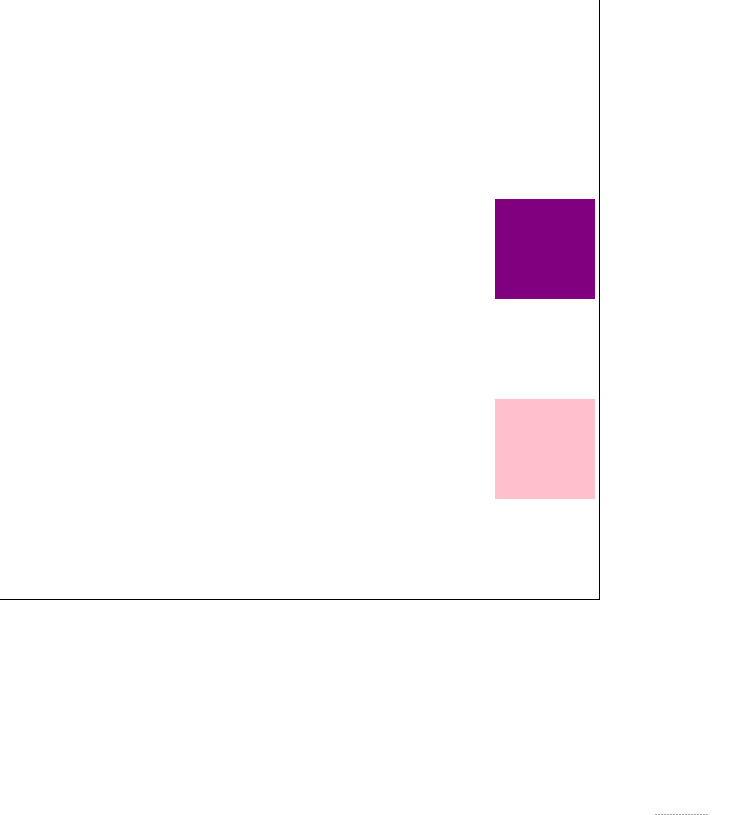## 二、Canvas的绘制功能

### 2.1 绘制矩形

``````if(ctx !== null) {
// 设置颜色
ctx.fillStyle = 'green'
// 填充矩形
ctx.fillRect(100, 100, 300, 50)
}
``````

``````if (ctx !== null) {
// 设置颜色
ctx.strokeStyle = 'red'
// 绘制矩形
ctx.strokeRect(300, 100, 100, 100)
}
``````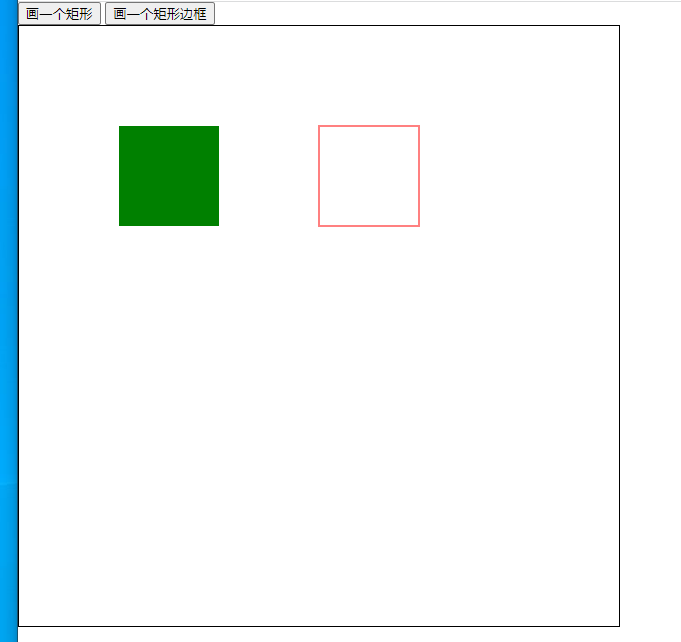``````// 擦除画布内容
btn3.onclick = () => {
if (ctx !== null) {
ctx.clearRect(0, 0, 600, 600)
}
}
``````

### 2.2 绘制路径

1. 需要设置路径的起点

2. 使用绘制命令画出路径

3. 封闭路径

4. 填充或者绘制已经封闭路径的形状

``````// 创建一个路径
ctx.beginPath()
// 1. 移动绘制点
ctx.moveTo(100, 100)
// 2. 描述行进路径
ctx.lineTo(200, 200)
ctx.lineTo(400, 180)
ctx.lineTo(380, 50)
// 3. 封闭路径
ctx.closePath();

// 4. 绘制这个不规则的图形
ctx.strokeStyle = 'red'
ctx.stroke()
ctx.fillStyle = 'orange'
ctx.fill()
``````1. 开始路径`ctx.beginPath()`
2. 移动绘制点`ctx.moveTo(x, y)`
3. 描述绘制路径`ctx.lineTo(x, y)`
4. 多次描述绘制路径`ctx.lineTo(x, y)`
5. 闭合路径`ctx.closePath()`
6. 描边`ctx.stroke()`
7. 填充`ctx.fill()`

`stroke()`: 通过线条来绘制图形轮廓。

`fill()`: 通过填充路径的内容区域生成实心的图形。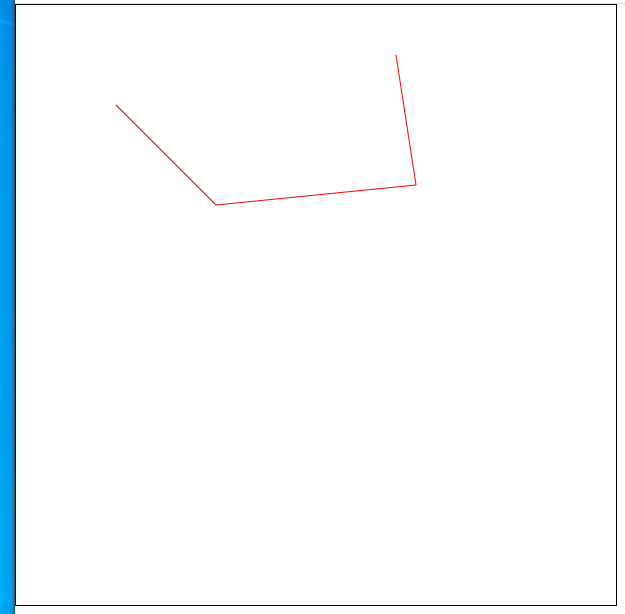### 2.3 绘制圆弧

`arc(x, y, radius, startAngle, endAngle, anticlockwise)`

``````// 创建一个路径
ctx.beginPath()
// 移动绘制点
// ctx.arc(200, 200, 100, 0, 2 * Math.PI, false)
ctx.arc(200, 200, 100, 0, 2 * 3.14, false)

ctx.stroke()
``````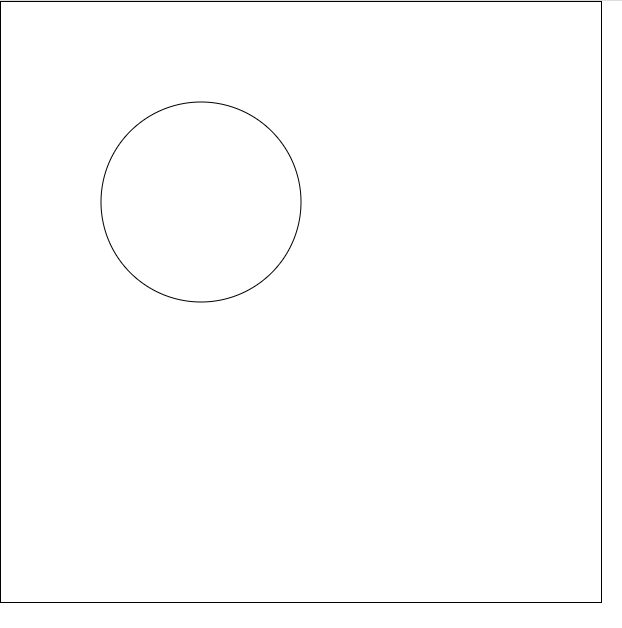### 2.4 炫彩小球

``````// 得到画布
const myCanvas: HTMLCanvasElement = document.getElementById("main_canvas") as HTMLCanvasElement

// 获取上下文
const ctx = myCanvas.getContext("2d")

class Ball {
color: string // 小球的颜色
r: number // 小球的半径
dx: number // 小球在x轴的运动速度/帧
dy: number // 小球在y轴的运动速度/帧
constructor(public x: number, public y: number) {
// 设置随机颜色
this.color = this.getRandomColor()
// 设置随机半径[1, 101)
this.r = Math.floor(Math.random() * 100 + 1)
// 设置x轴, y轴的运动速度(-5, 5)
this.dx = Math.floor(Math.random() * 10) - 5
this.dy = Math.floor(Math.random() * 10) - 5
}
// 随机颜色，最后返回的是类似'#3fe432'
getRandomColor(): string {
let allType = "0,1,2,3,4,5,6,7,8,9,a,b,c,d,e,f"
let allTypeArr = allType.split(',')
let color = '#'
for (let i = 0; i < 6; i++) {
let random = Math.floor(Math.random() * allTypeArr.length)
color += allTypeArr[random]
}
return color
}

// 渲染小球
render(): void {
if(ctx !== null) {
ctx.beginPath()
ctx.arc(this.x, this.y, this.r, 0, Math.PI * 2, false)
ctx.fillStyle=this.color
ctx.fill()
}
}

// 更新小球
update(): void {
// 小球的运动
this.x += this.dx
this.y += this.dy
this.r -= 0.5
// 如果小球的半径小于0了，从数组中删除
if(this.r <= 0) {
this.remove()
}
}

// 移除小球
remove(): void {
for(let i = 0; i < ballArr.length; i++) {
if(ballArr[i] === this) {
ballArr.splice(i, 1)
}
}
}
}
// 维护小球的数组
let ballArr: Ball[] = []

// canvas设置鼠标监听
ballArr.push(new Ball(event.offsetX, event.offsetY))
})

// 定时器进行动画渲染和更新
setInterval(function() {
// 动画的逻辑，清屏-更新-渲染
if(ctx !== null) {
ctx.clearRect(0, 0, myCanvas.width, myCanvas.height)
}
for(let i = 0; i < ballArr.length; i++) {
// 小球的更新和渲染
ballArr[i].update()
if(ballArr[i]) {
ballArr[i].render()
}

}
// 60 帧
}, 1000 / 60)
``````### 2.5 透明度

``````ctx.globalAlpha = 1
``````

### 2.6 小球连线

``````// 得到画布
const myCanvas: HTMLCanvasElement = document.getElementById("mycanvas") as HTMLCanvasElement

// 获取上下文
const ctx = myCanvas.getContext("2d")

// 设置画布的尺寸
myCanvas.width = document.documentElement.clientWidth - 30
myCanvas.height = document.documentElement.clientHeight - 30

class Ball {
x: number = Math.floor(Math.random() * myCanvas.width)
y: number = Math.floor(Math.random() * myCanvas.height)
r: number = 10
color: string = 'gray'
dx: number = Math.floor(Math.random() * 10) - 5
dy: number = Math.floor(Math.random() * 10) - 5
constructor() {
ballArr.push(this)
}

// 小球的渲染
render() {
if(ctx !== null) {
ctx.beginPath()
// 透明度
ctx.globalAlpha = 1
// 画小球
ctx.arc(this.x, this.y, this.r, 0, Math.PI * 2, false)
ctx.fillStyle = this.color
ctx.fill()
}
}
// 小球的更新
update() {
// 更新x
this.x += this.dx
// 纠正x
if(this.x <= this.r) {
this.x = this.r
} else if ( this.x >= myCanvas.width - this.r) {
this.x = myCanvas.width - this.r
}
// 更新y
this.y += this.dy
// 纠正y
if(this.y <= this.r) {
this.y = this.r
} else if ( this.y >= myCanvas.height - this.r) {
this.y = myCanvas.height - this.r
}
// 碰壁返回
if(this.x + this.r >= myCanvas.width || this.x - this.r <= 0) {
this.dx *= -1
}
if(this.y + this.r >= myCanvas.height || this.y - this.r <= 0) {
this.dy *= -1
}
}

}

// 小球数组
let ballArr: Ball[] = []

// 创建20个小球
for(let i = 0; i < 20; i++) {
new Ball()
}

// 定时器动画
setInterval(() => {
// 清除画布
if(ctx !== null) {
ctx.clearRect(0, 0, myCanvas.width, myCanvas.height)
}
// 小球渲染和更新
for(let i = 0; i < ballArr.length; i++) {
ballArr[i].render()
ballArr[i].update()
}
// 画线的逻辑
if(ctx !== null) {
for(let i = 0; i < ballArr.length; i++) {
for(let j = i + 1; j < ballArr.length; j++) {
let distance = Math.sqrt(Math.pow((ballArr[i].x - ballArr[j].x), 2) + Math.pow((ballArr[i].y -ballArr[j].y), 2))
if( distance <= 150) {
ctx.strokeStyle = '#000'
ctx.beginPath()
// 线的透明度，根据当前已经连线的小球的距离进行线的透明度设置
// 距离越近透明度越大，距离越远透明度越小
ctx.globalAlpha = 1 - distance / 150
ctx.moveTo(ballArr[i].x, ballArr[i].y)
ctx.lineTo(ballArr[j].x, ballArr[j].y)
ctx.closePath()
ctx.stroke()
}
}
}
}
}, 1000/60)
``````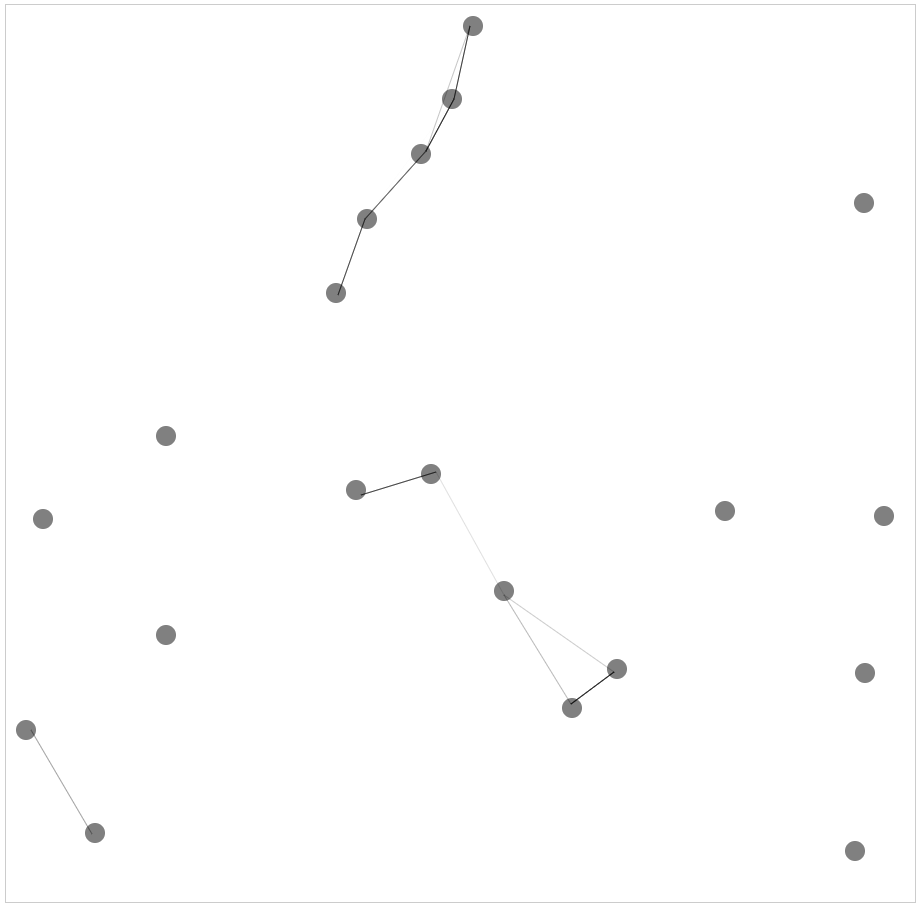### 2.7 线型

#### lineWidth

``````ctx.lineWidth = 1.0
``````#### lineCap

``````ctx.lineCap = "round";
``````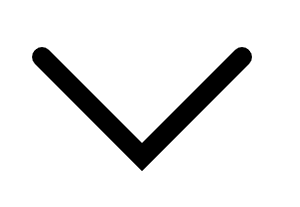#### lineJoin

``````ctx.lineJoin = "bevel";
``````
• `round`表示通过填充一个额外的，圆心在相连部分末端的扇形，绘制拐角的形状。 圆角的半径是线段的宽度。
• `bevel`表示在相连部分的末端填充一个额外的以三角形为底的区域， 每个部分都有各自独立的矩形拐角。
• `mitter`表示通过延伸相连部分的外边缘，使其相交于一点，形成一个额外的菱形区域。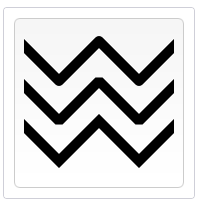#### setLineDash

``````ctx.setLineDash(segments);
``````
• `segments`是一个`Array`数组。一组描述交替绘制线段和间距（坐标空间单位）长度的数字。 如果数组元素的数量是奇数， 数组的元素会被复制并重复。例如， `[5, 15, 25]` 会变成 `[5, 15, 25, 5, 15, 25]`。数组内部是虚线的交替状态
``````// 得到画布
const myCanvas: HTMLCanvasElement = document.getElementById("mycanvas") as HTMLCanvasElement

// 获取上下文
const ctx = myCanvas.getContext("2d")

// 画布的尺寸
myCanvas.width = document.documentElement.clientWidth - 30
myCanvas.height = document.documentElement.clientHeight - 30

if(ctx !== null) {
ctx.setLineDash([15, 15]);
ctx.strokeRect(50,50, 90, 90)
ctx.setLineDash([15,10,2,10])
ctx.strokeRect(200,50, 90, 90)
}
``````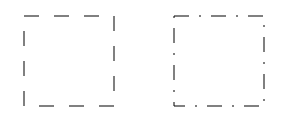#### lineDashOffset

``````ctx.lineDashOffset = value;
``````
• `value`偏移量是float精度的数字。 初始值为 `0.0`

### 2.8 文本

``````ctx.font = "30px 微软雅黑" // 空格前为文字大小，空格后为字体类型
// 第一个参数为文字内容，第二和第三个参数为文字绘制坐标，
// 第四个参数是可选参数，代表文字的最大宽度，如果字体宽度超过该值则压缩字体宽度
ctx.fillText("你好，世界！", 100, 100)
``````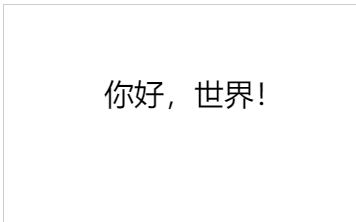``````ctx.textAlign = "left" || "right" || "center" || "start" || "end";
``````
• `left` : 文本左对齐。
• `right`: 文本右对齐。
• `center`: 文本居中对齐。
• `start`: 文本对齐界线开始的地方 （左对齐指本地从左向右，右对齐指本地从右向左）。
• `end`: 文本对齐界线结束的地方 （左对齐指本地从左向右，右对齐指本地从右向左）。

• 线性渐变：createLinearGradient 方法接受 4 个参数，表示图形渐变线的起点 (x1,y1) 与终点 (x2,y2)，渐变将沿着这条线向两边渐变。
``````ctx.createLinearGradient(x1, y1, x2, y2)
``````

``````let liner: CanvasGradient = ctx.createLinearGradient(0, 0, 100, 100)
ctx.fillStyle = liner
ctx.fillRect(10, 10, 100,100)
``````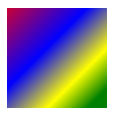``````let radial: CanvasGradient = ctx.createRadialGradient(100, 100, 0, 100, 100, 100)
ctx.arc(100, 100, 100, 0, Math.PI *2, false)
ctx.fill(
``````### 2.10 阴影

• `shadowOffsetX`: `shadowOffsetX``shadowOffsetY `用来设定阴影在 X 和 Y 轴的延伸距离，它们是不受变换矩阵所影响的。负值表示阴影会往上或左延伸，正值则表示会往下或右延伸，它们默认都为 `0`
• `shadowOffsetY`: `shadowOffsetX``shadowOffsetY `用来设定阴影在 X 和 Y 轴的延伸距离，它们是不受变换矩阵所影响的。负值表示阴影会往上或左延伸，正值则表示会往下或右延伸，它们默认都为 `0`
• `shadowBlur`: `shadowBlur` 用于设定阴影的模糊程度，其数值并不跟像素数量挂钩，也不受变换矩阵的影响，默认为 `0`
• `shadowColor`: `shadowColor` 是标准的 CSS 颜色值，用于设定阴影颜色效果，默认是全透明的黑色。
``````ctx.shadowOffsetX = 1 // 阴影左右偏离的距离
ctx.font ='30px 宋体'
ctx.fillText('你好，世界！', 100, 100)
``````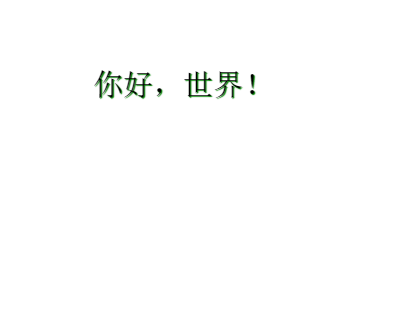## 三、使用图片

canvs中使用drawImage来绘制图片，主要是把外部的图片导入进来，绘制到画布上。

``````// 导入图片
if(ctx !== null) {
// 第一步是创建一个image元素
let image:HTMLImageElement = new Image()
// 用src设置图片的地址
image.src = "image/test1.png"
ctx.drawImage(image, 100, 100)
}
}
``````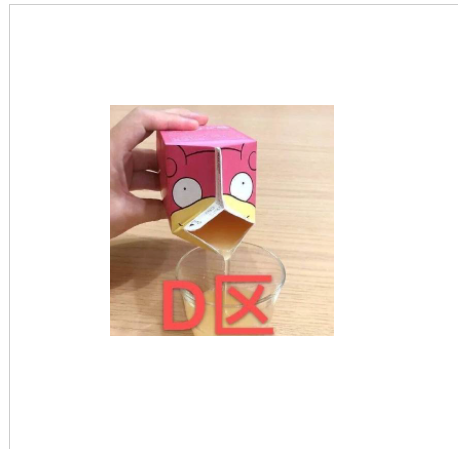``````ctx.drawImage(image, 100, 100)
``````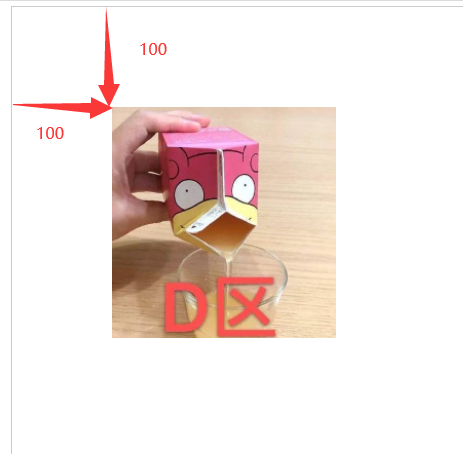``````ctx.drawImage(image, 100, 100, 50, 50)
``````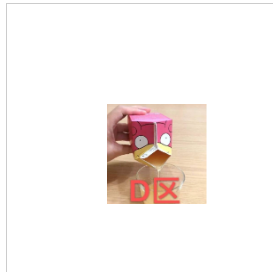``````// ctx.drawImage(image, sx, sy, sWidth, sHeight, dx, dy, dWidth, dHeight);
ctx.drawImage(image, 100, 300, 200, 200)
ctx.drawImage(image, 100, 100, 200, 200, 100, 100, 200, 200)
``````
• sx: 需要绘制到目标上下文中的，`image`的矩形（裁剪）选择框的左上角 X 轴坐标。
• sy: 需要绘制到目标上下文中的，`image`的矩形（裁剪）选择框的左上角 Y 轴坐标。
• sWidth: 需要绘制到目标上下文中的，`image`的矩形（裁剪）选择框的宽度。如果不说明，整个矩形（裁剪）从坐标的`sx``sy`开始，到`image`的右下角结束。
• sHeight: 需要绘制到目标上下文中的，`image`的矩形（裁剪）选择框的高度。
• dx: `image`的左上角在目标canvas上 X 轴坐标。
• dy: `image`的左上角在目标canvas上 Y 轴坐标。
• dWidth: `image`在目标canvas上绘制的宽度。 允许对绘制的`image`进行缩放。 如果不说明， 在绘制时`image`宽度不会缩放。
• dHeight: `image`在目标canvas上绘制的高度。 允许对绘制的`image`进行缩放。 如果不说明， 在绘制时`image`高度不会缩放。## 四、资源管理器## 4.1 获取对象中属性的长度

``````this.imgURL = {
'fengjing1':'./image/下载1.jpg',
'fengjing2':'./image/下载2.jpg',
'fengjing3':'./image/下载3.jpg',
'fengjing4':'./image/下载4.jpg',
'fengjing5':'./image/下载5.jpg',
}
``````

``````Object.keys(this.imgURL).length
``````

## 4.2 管理器的实现

``````interface StringOrImage {
// 定义了一个接口，该接口要求对象的属性是string或者是HTMLImageElement类型
[index: string]: string | HTMLImageElement
}

class Game {
dom: HTMLCanvasElement
ctx: CanvasRenderingContext2D | null
imgURL: StringOrImage
constructor() {
// 得到画布
this.dom = document.getElementById("mycanvas") as HTMLCanvasElement
// 获取上下文
this.ctx = this.dom.getContext("2d")
// 在属性中保存需要的图片地址
this.imgURL = {
// 'fengjing1':'./image/下载1.jpg',
// 'fengjing2':'./image/下载2.jpg',
// 'fengjing3':'./image/下载3.jpg',
// 'fengjing4':'./image/下载4.jpg',
// 'fengjing5':'./image/下载5.jpg',
'fengjing1':'https://gimg2.baidu.com/image_search/src=http%3A%2F%2Fimg.jj20.com%2Fup%2Fallimg%2F4k%2Fs%2F02%2F2109242332225H9-0-lp.jpg&refer=http%3A%2F%2Fimg.jj20.com&app=2002&size=f9999,10000&q=a80&n=0&g=0n&fmt=auto?sec=1651933471&t=34b40d339ce3bc4177afb393e7785575',
'fengjing2':'https://gimg2.baidu.com/image_search/src=http%3A%2F%2Ffile02.16sucai.com%2Fd%2Ffile%2F2014%2F0827%2Fc0c92bd51bb72e6d12d5b877dce338e8.jpg&refer=http%3A%2F%2Ffile02.16sucai.com&app=2002&size=f9999,10000&q=a80&n=0&g=0n&fmt=auto?sec=1651933483&t=453f28e751e0d54d70a2e3393e57b423',
'fengjing3':'https://gimg2.baidu.com/image_search/src=http%3A%2F%2Fimg.jj20.com%2Fup%2Fallimg%2F1113%2F032120114622%2F200321114622-4-1200.jpg&refer=http%3A%2F%2Fimg.jj20.com&app=2002&size=f9999,10000&q=a80&n=0&g=0n&fmt=auto?sec=1651933493&t=e9017fa69deb525312e214d2583a76d4',
'fengjing4':'https://pic.rmb.bdstatic.com/1530971282b420d77bdfb6444d854f952fe31f0d1e.jpeg',
'fengjing5':'https://gimg2.baidu.com/image_search/src=http%3A%2F%2Fimg.jj20.com%2Fup%2Fallimg%2Ftp01%2F1ZZQ214233446-0-lp.jpg&refer=http%3A%2F%2Fimg.jj20.com&app=2002&size=f9999,10000&q=a80&n=0&g=0n&fmt=auto?sec=1651933521&t=2cc050574824ec2761b539ab3a697522',
}
// 获取资源图片的总数
let imgCount = Object.keys(this.imgURL).length
// 计数器，记录的是加载完毕的数量
let count = 0
// 遍历imgURL对象获取每一个路径地址
for(let key in this.imgURL) {
// 备份每一张图片的地址
let src: string = this.imgURL[key] as string
// 创建一个图片
this.imgURL[key] = new Image();

// 判断图片是否加载完成，如果完成了，记数，如果加载完毕的数量和总数量相同了，则说明资源加载完毕
// 第一种方法，将值提取出去做类型缩小
let value = this.imgURL[key]
// 类型缩小成HTMLImageElement类型
if(typeof value !== 'string') {
value.src = src
// 增加计数器
count++
if(this.ctx !== null) {
// 清屏
this.ctx.clearRect(0, 0, 600, 600)
this.ctx.font = '16px Arial'
this.ctx.fillText("图片已经加载:" + count +" / " + imgCount, 50, 50)
// 判断图片是否加载完毕,如果加载完毕了再开始显示
if(count === imgCount) {
this.start()
}
}
}
}

// 第二种方法，使用as直接断言成HTMLImageElement
//(this.imgURL[key] as HTMLImageElement).src = src

}
}
start() {
if(this.ctx !== null) {
// 清屏
this.ctx.clearRect(0, 0, 600, 600)
let startX = 0
let startY = 0
for(let key in this.imgURL) {
this.ctx.drawImage(this.imgURL[key] as HTMLImageElement, startX, startY, 100, 100)
startX += 100
startY += 100
}
}
}
}

new Game()
``````

## 五、变形

canvas是可以进行变形的，但是变形的不是元素，而是ctx，ctx就是整个画布的渲染区域，整个画布在变形，我们需要在画布变形前进行保存和恢复：

• `save()`：保存画布（canvas）的所有状态。
• `restore()`：save 和 restore 方法是用来保存和恢复 canvas 状态的，都没有参数。Canvas 的状态就是当前画面应用的所有样式和变形的一个快照。

Canvas状态存储在栈中，每当`save()`方法被调用后，当前的状态就被推送到栈中保存。一个绘画状态包括：

``````// 得到画布
const myCanvas: HTMLCanvasElement = document.getElementById('mycanvas') as HTMLCanvasElement
// 获得上下文
const ctx = myCanvas.getContext('2d')

if (ctx !== null) {
ctx.fillRect(0, 0, 150, 150);   // 使用默认设置绘制一个矩形
ctx.save();                  // 保存默认状态

ctx.fillStyle = '#09F'       // 在原有配置基础上对颜色做改变
ctx.fillRect(15, 15, 120, 120); // 使用新的设置绘制一个矩形

ctx.save();                  // 保存当前状态
ctx.fillStyle = '#FFF'       // 再次改变颜色配置
ctx.globalAlpha = 0.5;
ctx.fillRect(30, 30, 90, 90);   // 使用新的配置绘制一个矩形

ctx.restore();               // 重新加载之前的颜色状态
ctx.fillRect(45, 45, 60, 60);   // 使用上一次的配置绘制一个矩形

ctx.restore();               // 加载默认颜色配置
ctx.fillRect(60, 60, 30, 30);   // 使用加载的配置绘制一个矩形
}
``````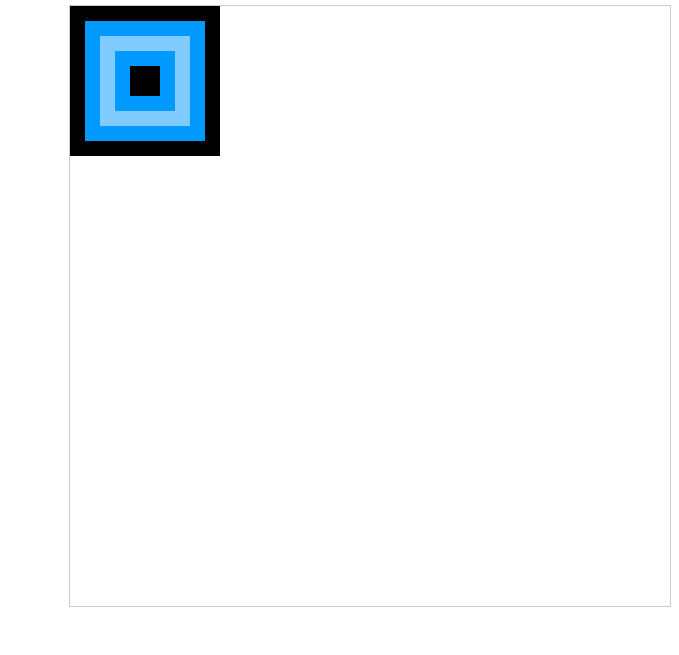### 5.1 移动translate

`translate(x, y)`: `translate `方法接受两个参数。x 是左右偏移量，y 是上下偏移量。

``````// 得到画布
const myCanvas: HTMLCanvasElement = document.getElementById('mycanvas') as HTMLCanvasElement
// 获得上下文
const ctx = myCanvas.getContext('2d')

if (ctx !== null) {
// 保存
ctx.save()
ctx.translate(50, 50)
ctx.fillRect(0, 0, 120, 120)
// 恢复
ctx.restore()
// 渲染位置是没有存档之前的位置
ctx.fillRect(120, 300, 120, 120)
}
``````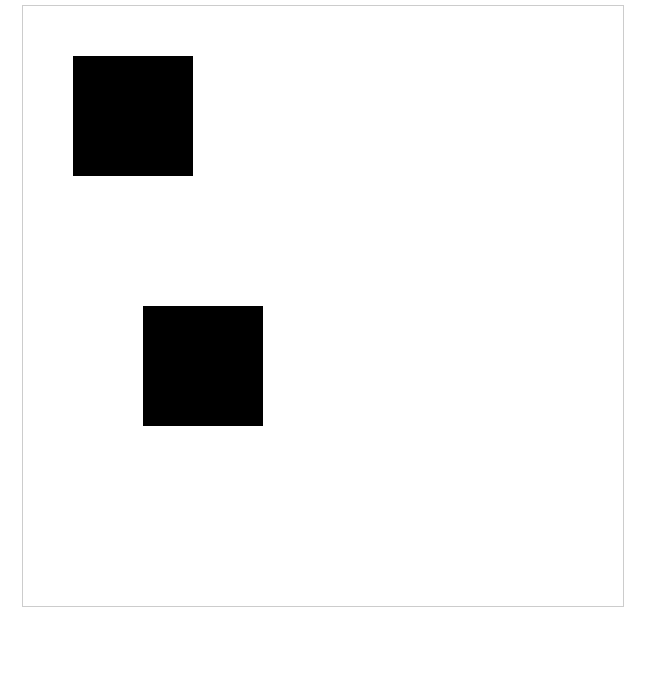### 5.2 旋转 rotate

`rotate(angle)`这个方法只接受一个参数：旋转的角度(angle)，它是顺时针方向的，以弧度为单位的值。

### 5.3 缩放 scale

`scale(x, y)`: `scale ` 方法可以缩放画布的水平和垂直的单位。两个参数都是实数，可以为负数，x 为水平缩放因子，y 为垂直缩放因子，如果比1小，会缩小图形， 如果比1大会放大图形。默认值为1， 为实际大小。

### 5.4 变形 transform

`transform(a, b, c, d, e, f)`

`a (m11)`: 水平方向的缩放;

`b(m12)`: 竖直方向的倾斜偏移;

`c(m21)`: 水平方向的倾斜偏移;

`d(m22)`: 竖直方向的缩放;

`e(dx)`: 水平方向的移动;

`f(dy)`: 竖直方向的移动.

``````// 得到画布
const myCanvas: HTMLCanvasElement = document.getElementById('mycanvas') as HTMLCanvasElement
// 获得上下文
const ctx = myCanvas.getContext('2d')

if (ctx !== null) {
// 保存
ctx.save()
ctx.transform(0.5, 0, 1, 0.5, 100, 100)
ctx.fillRect(0, 0, 100,100)
// 恢复
ctx.restore()
// 渲染位置是没有存档之前的位置
ctx.fillRect(0, 200, 100, 100)

}
``````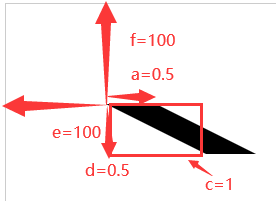### 5.5 滚动的车轮案例

• index.html
``````<!DOCTYPE html>
<html lang="en">

<meta charset="UTF-8">
<meta http-equiv="X-UA-Compatible" content="IE=edge">
<meta name="viewport" content="width=device-width, initial-scale=1.0">
<title>canvas的变形-滚动的车轮</title>

<body>
<canvas id="mycanvas"width="1200" height="600" >
当前的浏览器版本不支持，请升级浏览器
</canvas>
<script src='./dist/canvas.js'></script>
</body>
</html>
``````
• 所需图片• canvas.ts
``````// 得到画布
const myCanvas: HTMLCanvasElement = document.getElementById('mycanvas') as HTMLCanvasElement
// 获得上下文
const ctx = myCanvas.getContext('2d')

if (ctx !== null) {
// 第一步是创建一个image元素
const image:HTMLImageElement = new Image()
// 用src设置图片的地址
image.src = "image/汽车车轮.png"
// 定时器
// 旋转的度数
let deg = 0
// 位置
let x= -100

setInterval(() => {
// 清除画布
ctx.clearRect(0, 0, myCanvas.width, myCanvas.height)
deg += 0.1
x += 5
if(x >= myCanvas.width - 100) {
x = -100
}
// 备份
ctx.save()
// 平移, 目前我们的原点为(100,300)
ctx.translate(x, 300)
// 旋转,因为旋转始终在canvas的原点，所以我们得用translate改变原点。
ctx.rotate(deg)
// 我们得让车轮的中心处于原点，所以我们需要在第一个和第二个参数各为第三和第四个参数的一半然后再加负号
ctx.drawImage(image, -100, -100, 200, 200)
// 恢复
ctx.restore()
}, 1000/60)
}
}
``````
• 整体架构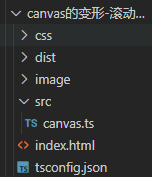• 实现的效果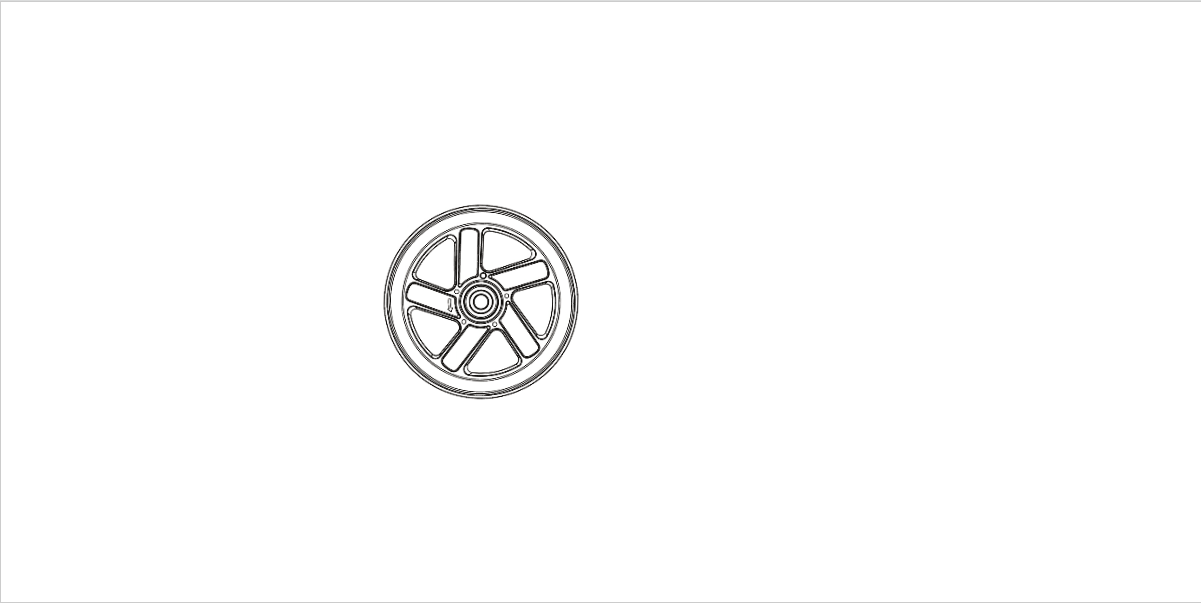## 六、合成与裁剪

``````// 得到画布
const myCanvas: HTMLCanvasElement = document.getElementById('mycanvas') as HTMLCanvasElement
// 获得上下文
const ctx = myCanvas.getContext('2d')

if (ctx !== null) {
ctx.fillStyle = 'skyblue'
ctx.fillRect(100, 100, 100, 100)
ctx.fillStyle = 'deeppink'
ctx.beginPath()
ctx.arc(200, 200, 60, 0, 7,false)
ctx.fill()
}
``````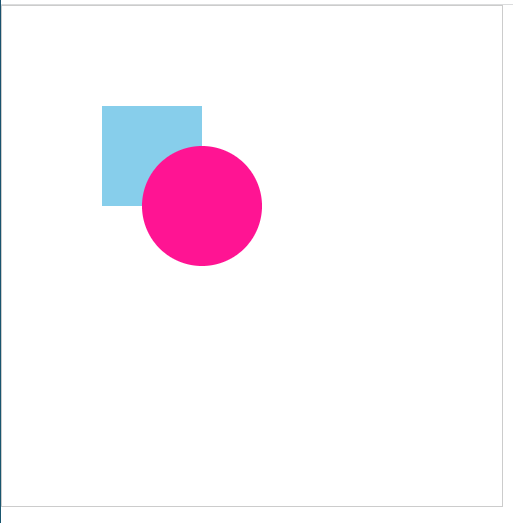### 6.1 globalCompositeOperation

`globalCompositeOperation = type`

``````// 得到画布
const myCanvas: HTMLCanvasElement = document.getElementById('mycanvas') as HTMLCanvasElement
// 获得上下文
const ctx = myCanvas.getContext('2d')

if (ctx !== null) {
ctx.fillStyle = 'skyblue'
ctx.fillRect(100, 100, 100, 100)
ctx.globalCompositeOperation= 'destination-over'
ctx.fillStyle = 'deeppink'
ctx.beginPath()
ctx.arc(200, 200, 60, 0, 7,false)
ctx.fill()
}
``````### 6.2 裁剪路径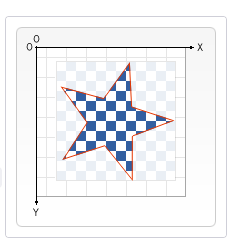`clip()`: 将当前正在构建的路径转换为当前的裁剪路径。默认情况下，canvas 有一个与它自身一样大的裁切路径（也就是没有裁切效果）。

### 6.3 刮刮乐案例

• index.html
``````<!DOCTYPE html>
<html lang="en">

<meta charset="UTF-8">
<meta http-equiv="X-UA-Compatible" content="IE=edge">
<meta name="viewport" content="width=device-width, initial-scale=1.0">
<title>canvas实现刮刮乐</title>

<body>
<div>
特等奖
<canvas width="250" height="60" id="mycanvas">
当前的浏览器版本不支持，请升级浏览器
</canvas>
</div>
<script src='./dist/canvas.js'></script>
</body>
</html>
``````
• index.css
``````div {
border: 1px solid #000;
width: 250px;
height: 60px;
font-size: 40px;
line-height: 60px;
text-align: center;
position: relative;
user-select: none;
}

canvas {
position: absolute;
left: 0;
top: 0;
}
``````
• canvas.ts
``````// 得到画布
const myCanvas: HTMLCanvasElement = document.getElementById('mycanvas') as HTMLCanvasElement
// 获得上下文
const ctx = myCanvas.getContext('2d')

if (ctx !== null) {
ctx.fillStyle = '#333'
ctx.fillRect(0, 0, 250, 60)
// 设置新画上的元素，实际上就是擦除之前的元素
ctx.globalCompositeOperation = 'destination-out'

const func = (event:any) => {
// 画图
ctx.beginPath()
ctx.arc(event.offsetX, event.offsetY,10, 0, Math.PI * 2,false)
ctx.fill()
}
// 按下
myCanvas.onmousedown = () => {
// 添加鼠标移动事件
}
// 松开
myCanvas.onmouseup = () => {
// 删除鼠标移动事件
myCanvas.removeEventListener('mousemove', func)
}
}
``````
• 实现效果